Module 3 - Answers Lesson 1 Answer 1 3.1.1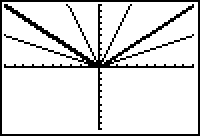The graph of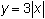is a vertical stretch of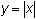by a factor of 3. The graph of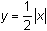is a vertical shrink (or compression) of the graph ofby a factor of 1/2.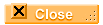Answer 2 3.1.2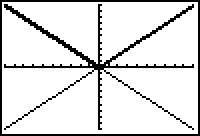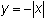is a reflection across the x-axis of.Lesson 2 Answer 1 3.2.1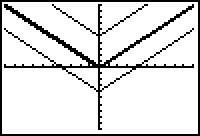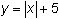is a vertical shift ofup 5.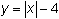is a vertical shift ofdown 4.Answer 2 3.2.2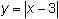produces a horizontal shift ofto the right 3 units.Answer 3 3.2.3 The graph of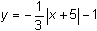is the graph of: Reflected about the x-axis. Vertically compressed by a factor of 1/3. Shifted left 5. Shifted down 1.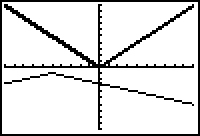Lesson 3 Answer 1 3.3.1 If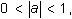the transformed graph will be a vertical shrink. If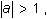the transformed graph will be a vertical stretch. If a < 0, the transformed graph will be a reflection across the x-axis.Answer 2 3.3.2 According to the table the investment will have grown to approximately \$1418.52 in six years.Answer 3 3.3.3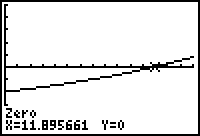The investment will double in approximately 12 years, which agrees with the solution found from the table of values.Answer 4 3.3.4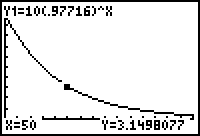About 3.15 grams remain after 50 days.Answer 5 3.3.5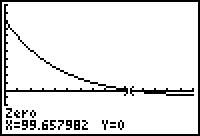There will be 1 gram remaining after about 100 days.Answer 6 3.3.6 Y2 = log(2x) appears to be a vertical shift of Y1 = log(x).Answer 7 3.3.7 The third graph suggests that the difference in the first two functions is a constant. In other words: Y2 – Y1 = C so Y2 = Y1 + C. This last equation supports the conjecture that Y2 is a vertical shift of Y1.Answer 8 3.3.8 For positive values of a and x, log(ax) = log(x) + log(a).Lesson 4 Answer 1 3.4.1 Make sure that the transformed regression equation is stored in Y2. In the TBLSET editor, set TblStart = 0 and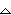Tbl = 10.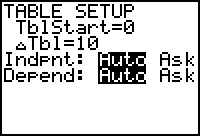Display the table by pressing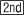[TABLE].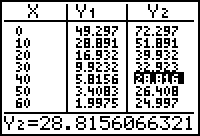The x-value that yields an output close to 30 is x = 40. So the transformed regression equation predicts that the water will cool to approximately 30°C in a bit less than 40 minutes, which corresponds well with the data.Self Test Answer 1 Any ordering of these 4 transformations will result in the correct graph as long as the vertical stretch and reflection are done before the vertical shift. Reflectacross the x-axis Vertical stretch by factor of 2 Shift left 1 Shift down 3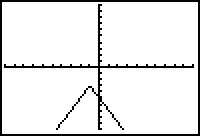Answer 2 The investment will double in about 10.2 years Answer 3 x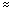222.4 Answer 4 Y2 is a vertical shift of Y1 downward and the shift is equal to log(2). Answer 5 y = 64(0.93)x + 23©Copyright 2007 All rights reserved. | Trademarks | Privacy Policy | Link Policy